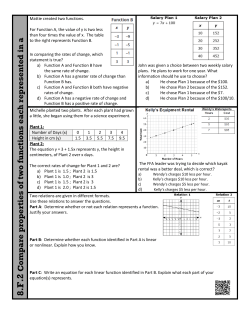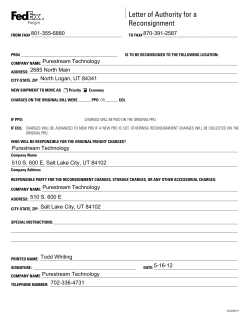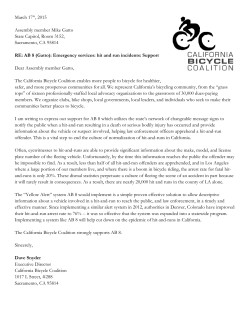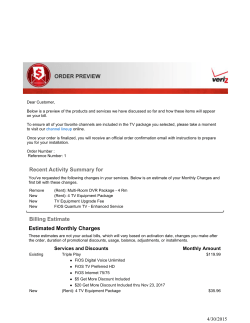# Name ____________________________________________ 6-3: Graphs in Real-World Situations Algebra Homework

```Name ____________________________________________ 6-3: Graphs in Real-World Situations
Homework
Algebra
1. Graph the function f x = 5 + 3x using a table of values.
()
()
f x = 5 + 3x
x
y
2. State the domain and range of the function in #1.
Stella and Julius are attending the local county fair. The parking will cost \$5 and admission is \$3
per person. The cost for x people is given by the function f x = 5 + 3x .
()
3. Using the graph from #1, determine the reasonable domain and range.
A banquet hall charges \$15 per person plus a \$100 setup fee. The cost for x people is given by
the function f x = 100 + 15x .
()
4. What are the reasonable domain and range?
For a function that models a real-world situation, the dependent variable y represents a person’s
height.
5. What is a reasonable range? Explain.
A tour company charges \$25 to hire a tour director plus \$75 per tour member. The total cost for a
group of x people is given by f x = 25 + 75x .
()
6. What is the reasonable domain? Explain.
Talk the Talk Cellular charges a base rate of \$20 per month for unlimited texts pus \$0.15 per
minute of talk time. The monthly cost for x minutes is given by f x = 20 + 0.15x .
()
7. Make sense of problems. What is the independent variable and what is the dependent
variable? Explain how you know.
8. What are the reasonable domain and range? Explain.
Alex has a bicycle that he rented from Fred’s Bicycle Shop. The bicycle rental charge is \$20 a day
plus \$0.30 per mile traveled. The cost for x miles traveled is given by the function
f x = 20 + 0.30 x .
()
9. What is a reasonable domain and range?
Pat’s Power Wash charges \$2.00 per car to cover the cost of cleaning supplies, plus \$0.50 per
minute for the use of water sprayers and vacuums.
10. Write a function to represent the total cost for x minutes of use of the water sprayer and
vacuums.
11. What is a reasonable domain and range?
```# Comp are prop erties o f two fun ctions each repre sented in a differe# Letter of Authority for a Reconsignment 801-355-6880 870-391-2587# The following descriptions will provide information about# March 17th, 2015 Assembly member Mike Gatto State Capitol# Choose a category. You will be given the answer. question.